# How to set, clear and toggle a single bit of a number in JavaScript?

• Last Updated : 28 Jan, 2020

Given a JavaScript number and the task is to set, clear and toggle a single bit of any number. Here are few approaches discussed with the help of JavaScript.

1. Setting the bit:
• Get the position of the bit to be set(starts from right, first bit is at 0 position), Ex. (bitSet = 3)
• Get the mask by mask = 1 << setBit, this mask is helpful in setting, clearing as well in toggling the bit.
• Use OR operator to set the particular bit.

Example: This example uses the approach discussed above.

 ` ` `<``html``> ` ` `  `<``head``> ` `    ``<``title``> ` `        ``Set, clear and toggle a single bit of a number. ` `    `` ` ` ` ` `  `<``body` `style``=``"text-align:center;"``> ` `    ``<``h1` `style``=``"color: green"``>   ` `            ``GeeksForGeeks   ` `        `` ` `    ``<``p` `id``=``"GFG_UP"` `style``=``"font-size: 20px; font-weight: bold;"``> ` `    `` ` `    ``<``button` `onclick``=``"gfg_Run()"``> ` `        ``Click Here ` `    `` ` `    ``<``p` `id``=``"GFG_DOWN"` `style``=``"color:green;"``> ` `    `` ` `    ``<``script``> ` `        ``var el_up = document.getElementById("GFG_UP"); ` `        ``var el_down = document.getElementById("GFG_DOWN"); ` `        ``var n = 6; ` `        ``var setBit = 3; ` `        ``el_up.innerHTML = "Click on the button to set the " ` `        ``+ setBit +  ` `          ``" bit of the number.<``br``>Number - " + n ` ` `  `        ``function gfg_Run() { ` `            ``var mask = 1 << ``setBit``; ` `            ``n` `= n | mask; ` `            ``el_down.innerHTML` `= n; ` `        ``} ` `    `` ` ` ` ` `  ` `

Output:

• Before clicking on the button: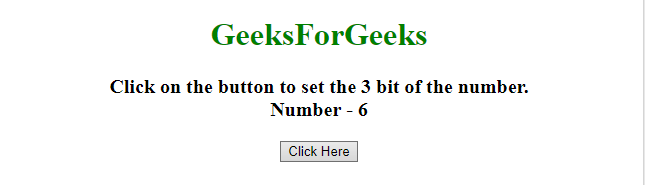• After clicking on the button: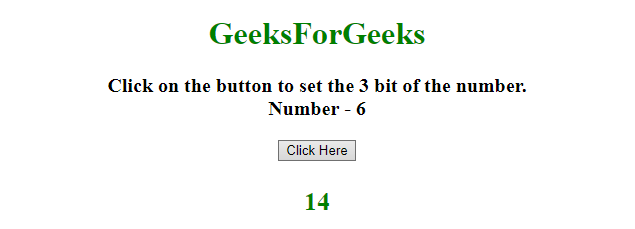2. Clearing the bit:
• Get the position of the bit to be clear(starts from right, first bit is at 0 position), Ex. (bitSet = 3)
• Use AND operator with negation of mask to clear the particular bit.

Example: This example uses the approach discussed above.

 ` ` `<``html``> ` ` `  `<``head``> ` `    ``<``title``> ` `        ``Set, clear and toggle a single bit of a number. ` `    `` ` ` ` ` `  `<``body` `style``=``"text-align:center;"``> ` `    ``<``h1` `style``=``"color: green"``>   ` `            ``GeeksForGeeks   ` `        `` ` `    ``<``p` `id``=``"GFG_UP"``> ` `    `` ` `    ``<``button` `onclick``=``"gfg_Run()"``> ` `        ``Click Here ` `    `` ` `    ``<``p` `id``=``"GFG_DOWN"` `style``=``"color:green;"``> ` `    `` ` `    ``<``script``> ` `        ``var el_up = document.getElementById("GFG_UP"); ` `        ``var el_down = document.getElementById("GFG_DOWN"); ` `        ``var n = 6; ` `        ``var setBit = 2; ` `        ``el_up.innerHTML = ` `          ``"Click on the button to clear the "  ` `        ``+ setBit + ` `          ``" bit of the number.<``br``>Number - " + n ` ` `  `        ``function gfg_Run() { ` `            ``var mask = 1 << ``setBit``; ` `            ``n &= ~mask; ` `            ``el_down.innerHTML` `= n; ` `        ``} ` `    `` ` ` ` ` `  ` `

Output:

• Before clicking on the button: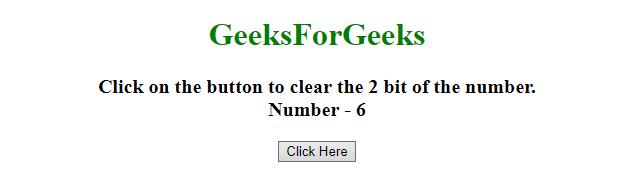• After clicking on the button: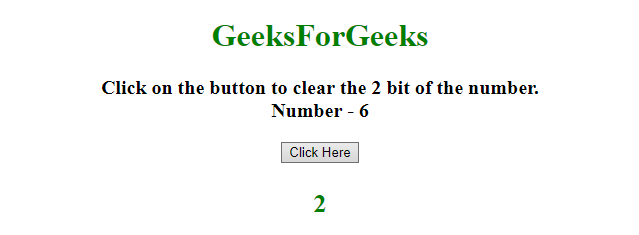3. Toggling the bit:
• Get the position of the bit to be toggle(starts from right, first bit is at 0 position), Ex. (bitSet = 3)
• Use XOR operator to toggle the particular bit.

Example: This example uses the approach discussed above.

 ` ` `<``html``> ` ` `  `<``head``> ` `    ``<``title``> ` `        ``Set, clear and toggle a single bit of a number. ` `    `` ` ` ` ` `  `<``body` `style``=``"text-align:center;"``> ` `    ``<``h1` `style``=``"color: green"``>   ` `            ``GeeksForGeeks   ` `        `` ` `    ``<``p` `id``=``"GFG_UP"``> ` `    `` ` `    ``<``button` `onclick``=``"gfg_Run()"``> ` `        ``Click Here ` `    `` ` `    ``<``p` `id``=``"GFG_DOWN"` `style``=``"color:green;"``> ` `    `` ` `    ``<``script``> ` `        ``var el_up = document.getElementById("GFG_UP"); ` `        ``var el_down = document.getElementById("GFG_DOWN"); ` `        ``var n = 6; ` `        ``var setBit = 3; ` `        ``el_up.innerHTML =  ` `          ``"Click on the button to toggle the "  ` `        ``+ setBit +  ` `          ``" bit of the number.<``br``>Number - " + n ` ` `  `        ``function gfg_Run() { ` `            ``var mask = 1 << ``setBit``; ` `            ``n ^= mask; ` `            ``el_down.innerHTML` `= n; ` `        ``} ` `    `` ` ` ` ` `  ` `

Output:

• Before clicking on the button: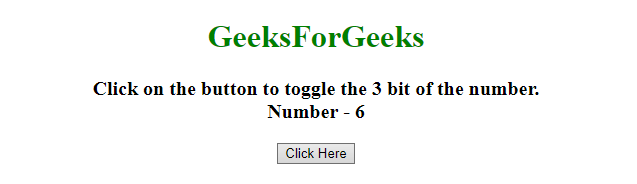• After clicking on the button: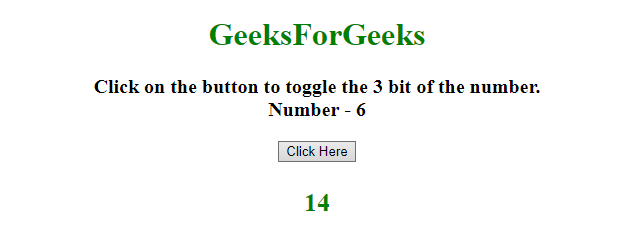My Personal Notes arrow_drop_up
Recommended Articles
Page :